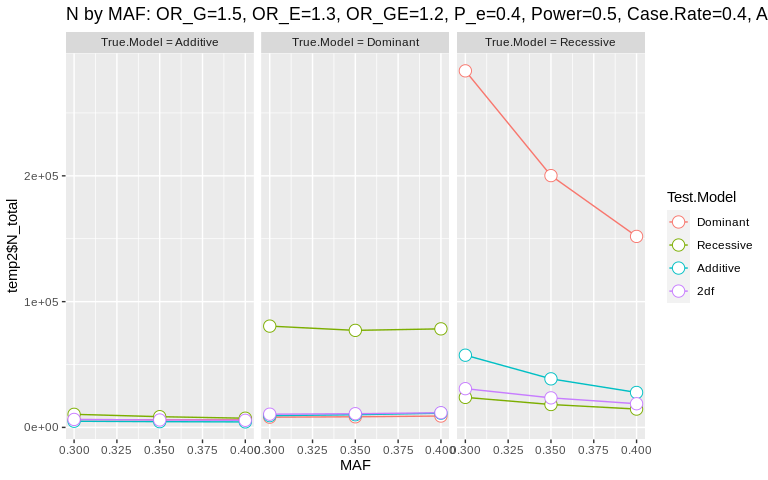# Introduction to genpwr

The genpwr package performs power and sample size calculations for genetic association studies, considering the impact of mis-specification of the genetic model. The user can specify the true genetic model, such as additive, dominant, and recessive, which represents the actual relationship between genotype and the outcome. The user also specifies a “Test” model, which indicates how the genetic effect will be coded for statistical testing. Options for test models include: additive, dominant, recessive and 2 degree of freedom (also called genotypic) tests.

The genpwr package allows the user to perform calculations for:

• Binary (case/control) or continuous outcome variables.

• Tests of gene and gene x environment interactions including both continuous and categorical environmental measurements.

genpwr is capable of calculating:

• sample size

• detectable effect size (or odds ratio in the case of a binary outcome variable)

• power

provided that two of the three above variables are entered into the appropriate genpwr function.

In addition to specifying of the three above variables (power, sample size, effect size), input variables include:

• Minor Allele Frequency

• Type 1 error rate, or “Alpha”

• “True” model type (recessive, dominant, additive)

• “Test” model type (recessive, dominant, additive, 2 degree of freedom)

• For binary outcomes / logistic regression models, either:
• Case rate
• The number of controls per case
• For continuous outcomes / linear regression models, the population standard deviation of the outcome.

Assuming an environmental exposure interaction term is to be tested:

• Population prevalence of environmental exposure for categorical environment variables or the standard deviation of the environmental exposure for continuous environment variables.

• Environmental exposure odds ratio (or effect size in the case of linear regression models)

• Environmental exposure / genetic variant interaction term odds ratio (or effect size in the case of linear regression models)

## Install genpwr

To install the package, first, you need to install the devtools package. You can do this from CRAN. Invoke R and then type:

install.packages("devtools")

library(devtools)

Install the package.

install_github("camillemmoore/Power_Genetics", subdir="genpwr")

library(genpwr)

## Examples

### Power Calculation for a Case Control Study

We calculate power to detect an odds ratio of 3 in a case control study with 400 subjects, including 80 cases and 320 controls (case rate of 20%) over a range of minor allele frequencies from 0.18 to 0.25. We calculate power for all possible combinations of true and test models, assuming an alpha of 0.05.

For a power calculation with a binary outcome and no gene/environment interaction, we use the following inputs:

pw <- genpwr.calc(calc = "power", model = "logistic", ge.interaction = NULL,
N=400, Case.Rate=0.2, k=NULL,
MAF=seq(0.18, 0.25, 0.01), OR=c(3),Alpha=0.05,
Test.Model=c("Dominant", "Recessive", "Additive", "2df"))

We look to see what the resulting data frame looks like:

head(pw)
#>    Test.Model True.Model  MAF OR N_total N_cases N_controls Case.Rate
#> 1    Dominant   Dominant 0.18  3     400      80        320       0.2
#> 3    Dominant   Additive 0.18  3     400      80        320       0.2
#> 5    Dominant  Recessive 0.18  3     400      80        320       0.2
#> 7    Dominant   Dominant 0.19  3     400      80        320       0.2
#> 9    Dominant   Additive 0.19  3     400      80        320       0.2
#> 11   Dominant  Recessive 0.19  3     400      80        320       0.2
#>    Power_at_Alpha_0.05
#> 1           0.98985513
#> 3           0.99730560
#> 5           0.08137351
#> 7           0.99040997
#> 9           0.99769410
#> 11          0.08615138

We then use the plotting function to plot these results

power.plot(pw)
#> Warning: Use of temp2$Power is discouraged. Use Power instead. #> Warning: Use of temp2$Test.Model is discouraged. Use Test.Model instead.
#> Warning: Use of temp2$Power is discouraged. Use Power instead. #> Warning: Use of temp2$Test.Model is discouraged. Use Test.Model instead.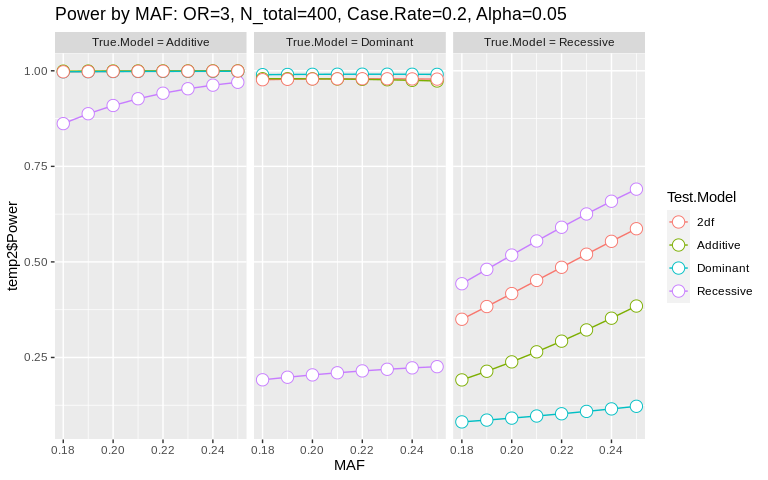A model with a continuous outcome can also be calculated:

pw <- genpwr.calc(calc = "power", model = "linear",
N=40, sd_y=4, k=NULL,
MAF=seq(0.18, 0.25, 0.01), ES=c(3),Alpha=0.05,
Test.Model=c("Dominant", "Recessive", "Additive", "2df"))

### Sample Size Calculation for a Case Control Study

ss <- genpwr.calc(calc = "ss", model = "logistic", ge.interaction = NULL,
OR=4, Case.Rate=0.4, k=NULL,
MAF=seq(0.3, 0.4, 0.02), Power=0.8, Alpha=0.05,
Test.Model=c("Dominant", "Recessive", "Additive", "2df"))

Plot:

ss.plot(ss)
#> Warning: Use of temp2$N_total is discouraged. Use N_total instead. #> Warning: Use of temp2$Test.Model is discouraged. Use Test.Model instead.
#> Warning: Use of temp2$N_total is discouraged. Use N_total instead. #> Warning: Use of temp2$Test.Model is discouraged. Use Test.Model instead.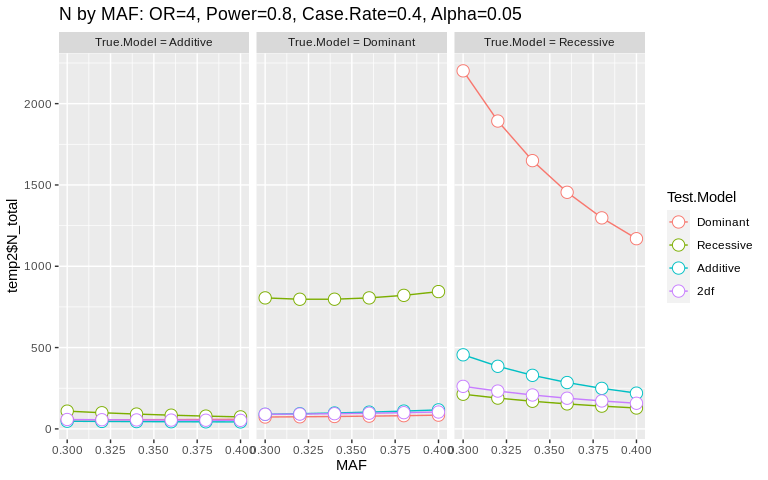### Detectable Odds Ratio Calculation for a Case Control Study

or <- genpwr.calc(calc = "es", model = "logistic", ge.interaction = NULL,
N=1000, Case.Rate=0.4, k=NULL,
MAF=seq(0.30, 0.4, 0.02), Power=0.4, Alpha=0.05,
True.Model="All", Test.Model="All")
or.plot(or)
#> Warning: Use of temp2$OR is discouraged. Use OR instead. #> Warning: Use of temp2$Test.Model is discouraged. Use Test.Model instead.
#> Warning: Use of temp2$OR is discouraged. Use OR instead. #> Warning: Use of temp2$Test.Model is discouraged. Use Test.Model instead.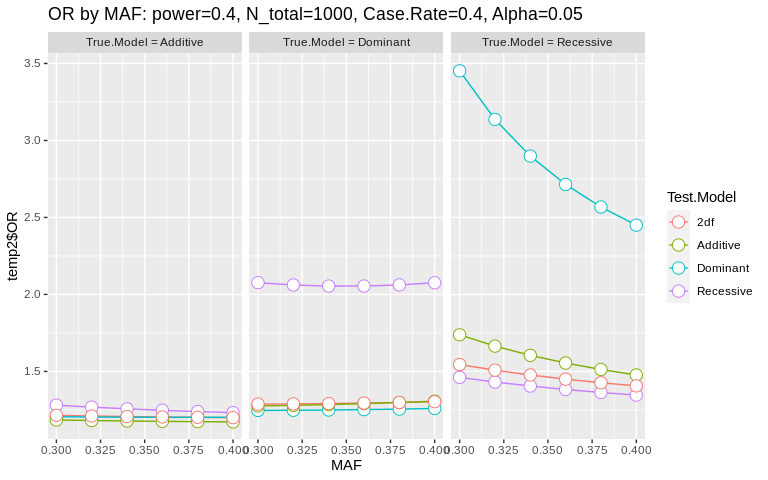### Power Calculation for a Case Control Study with a Gene x Environment Interaction

pec <- genpwr.calc(calc = "power", model = "logistic",
ge.interaction = "binary",
N=500, Case.Rate=0.3, MAF=seq(0.2,0.4,0.02), OR_G=3,
OR_E=3.5, OR_GE=4, P_e = 0.4,
Alpha=0.05, True.Model='All', Test.Model='All')
power.plot(pec)
#> Warning: Use of temp2$Power is discouraged. Use Power instead. #> Warning: Use of temp2$Test.Model is discouraged. Use Test.Model instead.
#> Warning: Use of temp2$Power is discouraged. Use Power instead. #> Warning: Use of temp2$Test.Model is discouraged. Use Test.Model instead.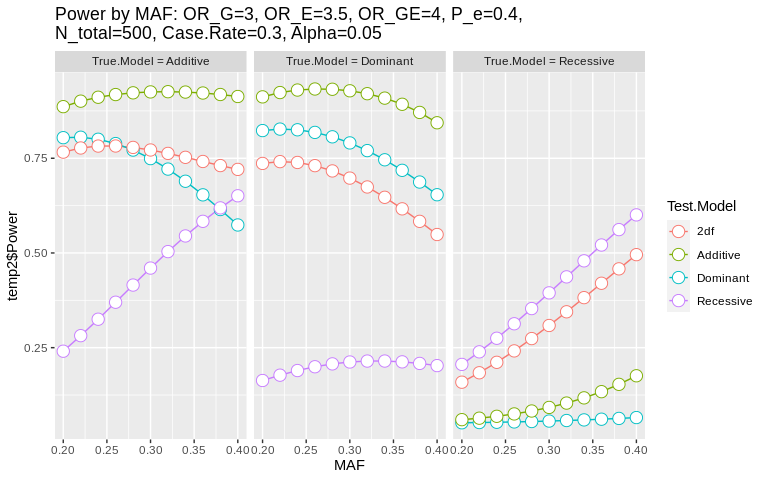Other options are available.

• linear model for continuous outcomes
pec <- genpwr.calc(calc = "power", model = "linear",
ge.interaction = "binary",
N=50, sd_y=3, MAF=seq(0.2,0.34,0.02), ES_G=3,
ES_E=1.5, ES_GE=2, P_e = 0.4,
Alpha=0.05, True.Model='All', Test.Model='All')
• continuous environment measurement
pec <- genpwr.calc(calc = "power", model = "logistic",
ge.interaction = "continuous",
N=500, Case.Rate=0.3, MAF=seq(0.25,0.31,0.02), OR_G=3,
OR_E=3.5, OR_GE=4, sd_e = 4,
Alpha=0.05, True.Model='All', Test.Model='All')

### Sample Size Calculation for a Case Control Study with a Gene x Environment Interaction

sse <- genpwr.calc(calc = "ss", model = "logistic", ge.interaction = "binary",
Power=0.5, Case.Rate=0.4, MAF=seq(0.3,0.4,0.05), OR_G=1.5,
OR_E=1.3, OR_GE=1.2, P_e = 0.4,
Alpha=0.05, True.Model="All", Test.Model="All")
ss.plot(sse)
#> Warning: Use of temp2$N_total is discouraged. Use N_total instead. #> Warning: Use of temp2$Test.Model is discouraged. Use Test.Model instead.
#> Warning: Use of temp2$N_total is discouraged. Use N_total instead. #> Warning: Use of temp2$Test.Model is discouraged. Use Test.Model instead.